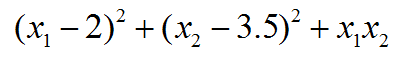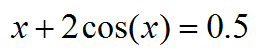# 无需导数的局部极小化算法NEWUOA在Fortran中的使用简例

## 1 NEWUOA简介

NEWUOA和其它极小化算法一样需要进行迭代，而且结果依赖于初猜。它不需要导数的好处很明显，很多情况函数是个黑箱，本身没有解析导数，或者解析导数代码很难写，如果用有限差分来计算导数，一方面昂贵，一方面还有数值精度层面的问题。根据Experimental Comparisons of Derivative Free Optimization Algorithms一文的对比（Google一搜就有），相对于流行的BFGS方法（假设梯度通过有限差分得到），多数情况下NEWUOA效率更高，即收敛到极小点所需要计算函数值的次数更少。NEWUOA的原理我就不在这里介绍了，大家可以看https://en.wikipedia.org/wiki/NEWUOA

Powell分享了他的Fortran77写的NEWUOA程序，后来有人写了Fortran95的wrapper（https://github.com/ralna/MJDP_software/tree/master/newuoa），我又进一步做了轻微修改使之用着更舒服，可以在这里下载：http://sobereva.com/attach/536/newuoa_module.f90。下面就基于这个文件，通过两个很简单的问题示例怎么使用NEWUOA方法解决实际问题，你会发现超级容易。

## 2 例：二元函数极小化PROGRAM test_newuoa
use newuoa_module
implicit real*8 (a-h,o-z)
real*8,allocatable :: X(:)
external CALFUN

nvar=2
allocate(X(nvar))
X(:)=0
call newuoa_min(CALFUN, X, RHOBEG=0.1D0, RHOEND=1D-6, IPRINT=2, MAXFUN=50000)
END PROGRAM

subroutine CALFUN(X,F)
real*8 :: X(:),F
F=(X(1)-2)**2+(X(2)-3.5D0)**2+X(1)*X(2)
end subroutine

New RHO = 1.0000D-02     Current number of function evaluations =    17
Least value of F =  3.918956916835460D+00
The corresponding X array is:
3.772896D-01   3.340358D+00

New RHO = 1.0000D-03     Current number of function evaluations =    22
Least value of F =  3.916670957635140D+00
The corresponding X array is:
3.356529D-01   3.331668D+00

New RHO = 1.0000D-04     Current number of function evaluations =    26
Least value of F =  3.916666676331397D+00
The corresponding X array is:
3.334374D-01   3.333242D+00

New RHO = 1.0000D-05     Current number of function evaluations =    30
Least value of F =  3.916666666692425D+00
The corresponding X array is:
3.333392D-01   3.333330D+00

New RHO = 1.0000D-06     Current number of function evaluations =    33
Least value of F =  3.916666666666715D+00
The corresponding X array is:
3.333336D-01   3.333333D+00

At the return from NEWUOA     Total times of function evaluations =    37
Least value of F =  3.916666666666666D+00
The corresponding X array is:
3.333333D-01   3.333333D+00

## 3 例：单变量求解PROGRAM test_newuoa
use newuoa_module
implicit real*8 (a-h,o-z)
real*8,allocatable :: X(:)
external :: CALFUN

nvar=1
allocate(X(nvar))
X(1)=0
call newuoa_min(CALFUN, X, RHOBEG=0.1D0, RHOEND=1D-6, IPRINT=1, MAXFUN=50000)

END PROGRAM

subroutine CALFUN(X,F)
real*8 :: X(:),F
F=abs(X(1)+2*cos(X(1))-0.5D0)
end subroutine

At the return from NEWUOA     Total times of function evaluations =    24
Least value of F =  2.144288913097370D-08
The corresponding X array is:
-8.379604D-01